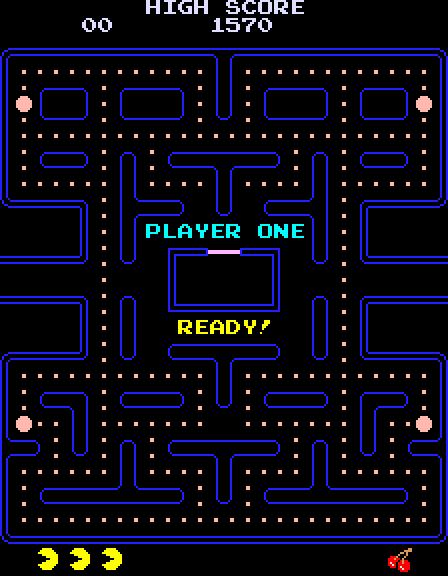# Computing $\pi$ using atoms

Everyone knows it's possible to compute $\pi$ by throwing darts at random but it's not so well known that you can calculate it by simulating an atom in a periodic box.

Remember Pac-Man? You could move the character all the way to the right of the maze and pop up at the left or down to the bottom and reappear at the top. The maze is akin to the flat torus $\T = \R^2 /\!\sim$, where $x \sim y$ if and only if $x_i = y_i \! \mod 2 \pi$ for $i = 1, 2$.If we fix a velocity $v \in \R^2$ and set in motion a particle originally at $x_0 \in \T$, then our particle follows the trajectory $x(t) = x_0 + t v$ forever.

Something interesting happens depending on how we choose $v = (v_1, v_2)$. If $\frac{v_1}{v_2}$ is a rational number, then the trajectory $x(t)$ is periodic.

However, if $\frac{v_1}{v_2}$ is irrational, then the set of visited points $\{ x(t) \, | \, t \ge 0 \}$ is dense in $\T$. In other words, the particle eventually gets arbitrarily close to any point of the torus without ever visiting twice the same spot.

We can now use this to measure $\pi$! Indeed, if $S \subset \T$ is a circle of radius $r = 1$, then \begin{equation*} \bar{A} = \lim_{T \to \infty} \frac{1}{T} \int_0^T \mathbb{1}_{S}(x(t)) \, \d t \end{equation*} is the fraction of the time that the particle spends inside $S$. It turns out that \begin{equation*} \pi = (4 \bar{A})^{-1} \end{equation*} as long as our initial velocity satisfies $\boldsymbol{\frac{v_1}{v_2} \not\in \mathbb{Q}}$.

Indeed, our approximations of $(4 \bar{A})^{-1}$ converge to $\pi$ as $T \to \infty$.

This works thanks to the ergodic theorem which, in the above setting, tells us that the time average $\bar{A}$ is equal to the expected value \begin{equation*} \mathbb{E}[\mathbb{1}_S] = \mathbb{P}(S), \end{equation*} with respect to the uniform probability distribution $\mathbb{P}$ on $\T$. The latter is simply the ratio of area of the circle $S$ to the area of the flat torus $\T$.

This can be seen as an instance of (Hamiltonian) Monte Carlo, but without randomness. Alternatively, we can regard it as an application of molecular dynamics to a gas particle in a 2D periodic box.

Published: November 15, 2022.